# 漫画：什么是冒泡排序？————— 当天上午 —————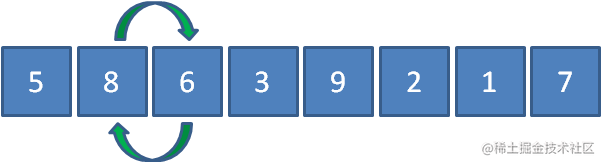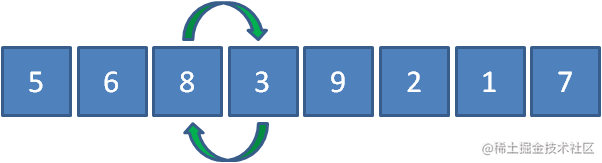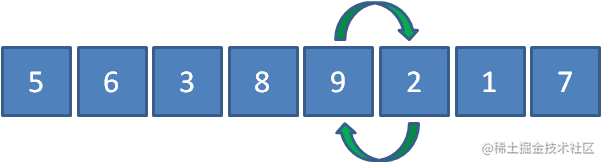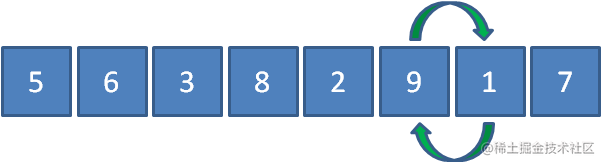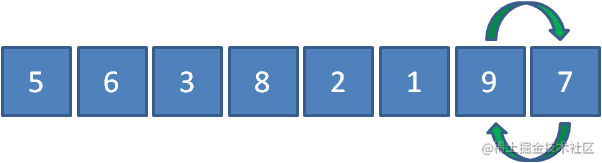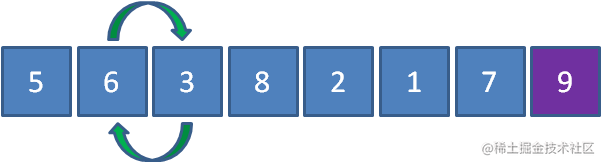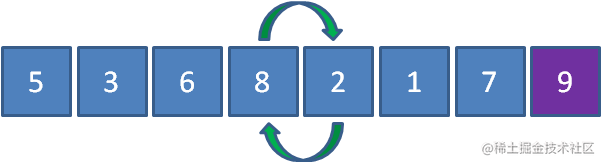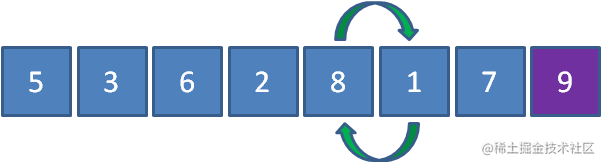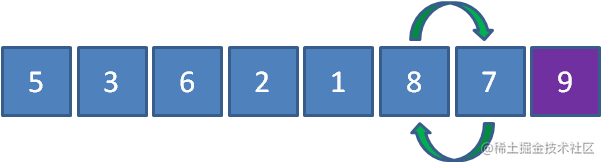public class BubbleSort {

``````private static void sort(int array[]){
int tmp  = 0;

for(int i = 0; i < array.length; i++){        for(int j = 0; j < array.length - i - 1; j++)        {            if(array[j] > array[j+1])            {                tmp = array[j];                array[j] = array[j+1];                array[j+1] = tmp;            }        }    }}public static void main(String[] args){    int[] array = new int[]{5,8,6,3,9,2,1,7};    sort(array);    System.out.println(Arrays.toString(array));}复制代码``````

}————————————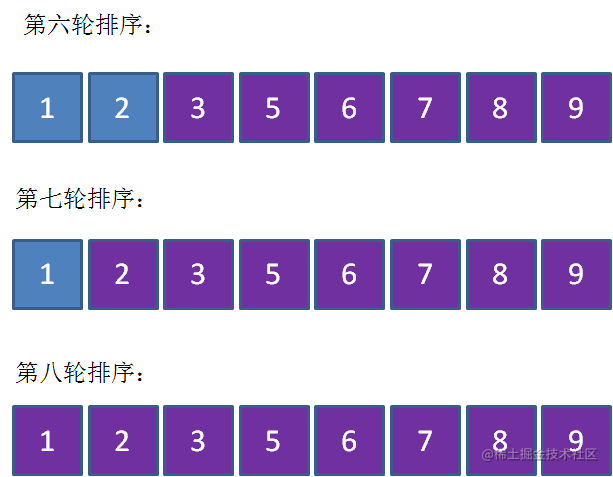public class BubbleSort {

``````private static void sort(int array[]){
int tmp  = 0;
for(int i = 0; i < array.length; i++)    {        //有序标记，每一轮的初始是true        boolean isSorted = true;        for(int j = 0; j < array.length - i - 1; j++)        {            if(array[j] > array[j+1])            {                tmp = array[j];                array[j] = array[j+1];                array[j+1] = tmp;                //有元素交换，所以不是有序，标记变为false                isSorted = false;            }        }        if(isSorted){            break;        }    }}public static void main(String[] args){    int[] array = new int[]{5,8,6,3,9,2,1,7};    sort(array);    System.out.println(Arrays.toString(array));}复制代码``````

}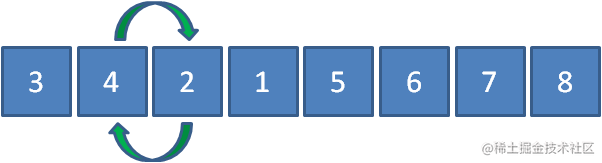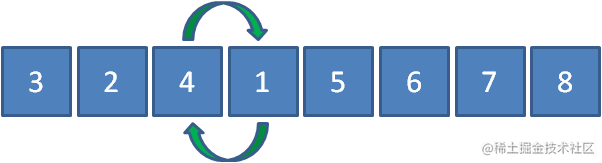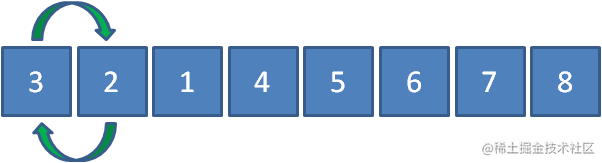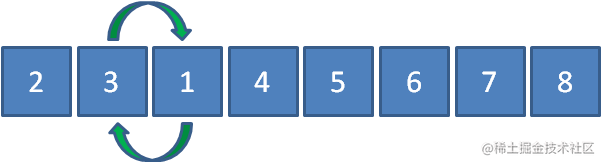public class BubbleSort {

``````private static void sort(int array[]){    int tmp  = 0;    //记录最后一次交换的位置    int lastExchangeIndex = 0;    //无序数列的边界，每次比较只需要比到这里为止    int sortBorder = array.length - 1;    for(int i = 0; i < array.length; i++)    {        //有序标记，每一轮的初始是true        boolean isSorted = true;        for(int j = 0; j < sortBorder; j++)        {            if(array[j] > array[j+1])            {                tmp = array[j];                array[j] = array[j+1];                array[j+1] = tmp;                //有元素交换，所以不是有序，标记变为false                isSorted = false;                //把无序数列的边界更新为最后一次交换元素的位置                lastExchangeIndex = j;            }        }        sortBorder = lastExchangeIndex;        if(isSorted){            break;        }    }}

public static void main(String[] args){    int[] array = new int[]{3,4,2,1,5,6,7,8};    sort(array);    System.out.println(Arrays.toString(array));}复制代码``````

}